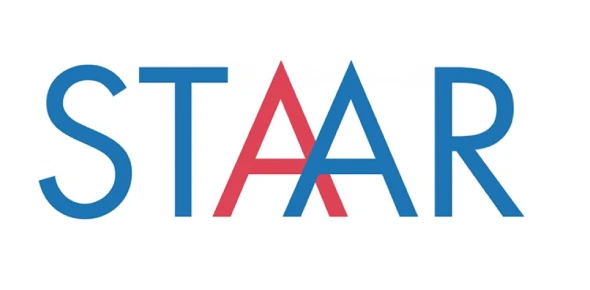# STAAR 7 TAKS Workout Quiz Lessons 1-9

Approved & Edited by ProProfs Editorial Team
At ProProfs Quizzes, our dedicated in-house team of experts takes pride in their work. With a sharp eye for detail, they meticulously review each quiz. This ensures that every quiz, taken by over 100 million users, meets our standards of accuracy, clarity, and engagement.
| Written by Mathdancer
M
Mathdancer
Community Contributor
Total Contribution - 3 | Total attempts - 1,620
Questions: 10 | Attempts: 66SettingsCovers the following skills.
Comparing & ordering
Integer Operations
Fractions,Decimals, Percent Conversions
Ratios, Rates
Square Roots
Single Digit divisor/Decimal Divident

• 1.

### At a Star of Texas rodeo, 26% of the cowboys wore black hats, 24% wore white hats, 22% wore other colored hats, and 28% wore helmets.  Which headwear was least popular?

• A.

Black hats

• B.

White hats

• C.

Hats of other colors

• D.

Helmets

C. Hats of other colors
Explanation
The least popular headwear is hats of other colors, as only 22% of the cowboys wore them.

Rate this question:

• 2.

### At Natural Bridge Caverns, the North Cavern is 180 feet below the surface.  The Fault Room is 230 feet below the surface.  What is the change in feet from the North Cavern to the Fault Room?

• A.

410 ft

• B.

50 ft

• C.

-50 ft

• D.

-410 ft

C. -50 ft
Explanation
The change in feet from the North Cavern to the Fault Room is -50 ft. This means that the Fault Room is 50 feet lower than the North Cavern.

Rate this question:

• 3.

### By what number do you divide 10 by to get -2?

• A.

-8

• B.

-5

• C.

5

• D.

8

B. -5
Explanation
To find the number by which 10 should be divided to get -2, we can use the formula: dividend ÷ divisor = quotient. In this case, the dividend is 10 and the quotient is -2. Rearranging the formula, we get: divisor = dividend ÷ quotient. Therefore, the divisor is 10 ÷ -2, which equals -5. Hence, the correct answer is -5.

Rate this question:

• 4.

### Which of the following shows equivalent fractions and decimals?

• A.

1/8 and 1.8

• B.

0.2 and 1/2

• C.

0.07 and 7/100

• D.

7/25 and 0.725

C. 0.07 and 7/100
Explanation
The correct answer is 0.07 and 7/100. This is because 0.07 can be written as a fraction by placing it over 100, resulting in 7/100. Both 0.07 and 7/100 represent the same value.

Rate this question:

• 5.

### Which group shows equivalent fractions, decimals and percents?

• A.

7/10, 0.7, 7%

• B.

1/8, 0.18, 18%

• C.

3/8, 0.375, 375%

• D.

2/5, 0.4, 40%

D. 2/5, 0.4, 40%
Explanation
The group that shows equivalent fractions, decimals, and percents is 2/5, 0.4, 40%. This is because when we convert 2/5 to a decimal, we get 0.4, and when we convert it to a percent, we get 40%. Therefore, all three representations are equivalent.

Rate this question:

• 6.

### Beth earned \$15 when she babysat for 3 hours.  What is the ratio of dollars to hours?

• A.

1:5

• B.

5:1

• C.

1:3

• D.

3:1

B. 5:1
Explanation
The ratio of dollars to hours is 5:1 because Beth earned \$15 for 3 hours of babysitting. This means that for every 5 dollars she earned, she babysat for 1 hour.

Rate this question:

• 7.

### The area of a square patio is 64 square feet. What is the width of the patio?

• A.

4 ft

• B.

8 ft

• C.

16 ft

• D.

32 ft

B. 8 ft
Explanation
The area of a square is found by multiplying the length of one side by itself. In this case, the area of the patio is given as 64 square feet. To find the width, we need to find the length of one side of the square. Taking the square root of 64 gives us 8, which represents the length of one side. Therefore, the width of the patio is 8 ft.

Rate this question:

• 8.

### Roger drove 192 miles in 4 hours.  What was the average speed for the trip?

• A.

768 mph

• B.

96 mph

• C.

48 mph

• D.

4 mph

C. 48 mph
Explanation
The average speed for the trip can be calculated by dividing the total distance traveled (192 miles) by the total time taken (4 hours). Therefore, the average speed for the trip is 48 mph.

Rate this question:

• 9.

### A package of 4 Brand A pens costs \$2.60.  A package of 10 Brand B pens costs \$6.00.  Compare the unit prices.

• A.

Brand A costs 5 cents more per pen

• B.

Brand A costs 6 cents more per pen

• C.

Brand B costs 6 cents more per pen.

• D.

The pens cost the same price.

A. Brand A costs 5 cents more per pen
Explanation
Brand A costs 5 cents more per pen compared to Brand B. This can be determined by calculating the unit price for each brand. For Brand A, the unit price is \$2.60 divided by 4 pens, which equals \$0.65 per pen. For Brand B, the unit price is \$6.00 divided by 10 pens, which equals \$0.60 per pen. Therefore, Brand A costs 5 cents more per pen than Brand B.

Rate this question:

• 10.

### Lydia has a piece of fabric that is 8.32 meters long.  She cuts it into 8 pieces of equal length.  How long is each piece of fabric?

• A.

14 meters

• B.

1.4 meters

• C.

1.14 meters

• D.

1.04 metersBack to top